How to Learn in 24 Hours?The Rapid Learning Movie

 Need Help? M-F: 9am-5pm(PST): Toll-Free: (877) RAPID-10 US Direct: (714) 692-2900 Int'l: 001-714-692-2900 24/7 Online Technical Support: The Rapid Support Center Secure Online Order:Need Proof? Testimonials by Our Users

 Rapid Learning Courses: MCAT in 24 Hours (2015-16) USMLE in 24 Hours (Boards) Chemistry in 24 Hours Biology in 24 Hours Physics in 24 Hours Mathematics in 24 Hours Psychology in 24 Hours SAT in 24 Hours ACT in 24 Hours AP in 24 Hours CLEP in 24 Hours DAT in 24 Hours (Dental) OAT in 24 Hours (Optometry) PCAT in 24 Hours (Pharmacy) Nursing Entrance Exams Certification in 24 Hours eBook - Survival Kits Audiobooks (MP3)

 Tell-A-Friend: Have friends taking science and math courses too? Tell them about our rapid learning system.Home »  Mathematics »  Introductory Statistics

Density Curves

 Topic Review on "Title": Mean of a Density Curve: think of a fulcrum balancing a density curve, the line inside the curve represents the mean.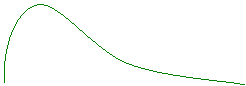If more weight is on the left of the curve than the right, the curve would dip on the left. If more weight is on the right of the curve than the left, the curve would dip on the right. Symmetric Curve: Mean and Median: the mean and median are the same for a symmetric density curve.Mean = Median Median Left Skewed Curve: Mean and Median: in a left skewed distribution, the mean is on the left closer to the tail of the distribution. The median is located at the center of the data.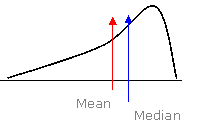Right Skewed Curve: Mean and Median In a right skewed distribution, the mean is on the right closer to the tail of the distribution. The median is located at the center of the data.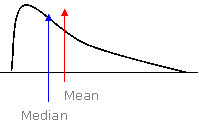Rapid Study Kit for "Title":
 Flash Movie Flash Game Flash Card Core Concept Tutorial Problem Solving Drill Review Cheat Sheet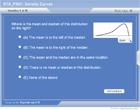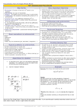"Title" Tutorial Summary : This tutorial shows the density curves and their properties. Many illustrative graphs are used to show you what density curve is, their shapes, and how to identify a density curve, etc. After this tutorial you will be able to identify a density curve, name the shape of the density curve, understand the importance of the area under the density curve and locate the mean and median of a density curve.

 Tutorial Features: Specific Tutorial Features: We use a lot of graphs in illustrating the different density curves and their shape and how to obtain their means, step by step. The way to balance density curve and the relationship between mean and median are also demonstrated in the examples. Series Features: Concept map showing inter-connections of new concepts in this tutorial and those previously introduced. Definition slides introduce terms as they are needed. Visual representation of concepts Animated examples—worked out step by step A concise summary is given at the conclusion of the tutorial.

 "Title" Topic List: Density Curve Mean of a Density Curve Median of a Density Curve

See all 24 lessons in Introductory Statistics, including concept tutorials, problem drills and cheat sheets:
Teach Yourself Introductory Statistics Visually in 24 Hours